Courses

# Test: Block Diagram Algebra

## 10 Questions MCQ Test Control Systems | Test: Block Diagram Algebra

Description
This mock test of Test: Block Diagram Algebra for Electrical Engineering (EE) helps you for every Electrical Engineering (EE) entrance exam. This contains 10 Multiple Choice Questions for Electrical Engineering (EE) Test: Block Diagram Algebra (mcq) to study with solutions a complete question bank. The solved questions answers in this Test: Block Diagram Algebra quiz give you a good mix of easy questions and tough questions. Electrical Engineering (EE) students definitely take this Test: Block Diagram Algebra exercise for a better result in the exam. You can find other Test: Block Diagram Algebra extra questions, long questions & short questions for Electrical Engineering (EE) on EduRev as well by searching above.
QUESTION: 1

### Consider the block diagram shown below: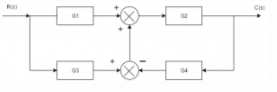If the transfer function of the system is given by T(s)=G1G2+G2G3/1+X. Then X is:

Solution:

Explanation: Use the technique of making two different block diagram by dividing two summers and use the approaches of shifting take off point and blocks.

QUESTION: 2

### For the block diagram given in the following figure, the expression of C/R is: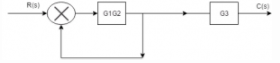Solution:

Explanation: Block diagram is being converted into signal flow graphs by considering each take off point as a node and each forward transfer function as forward gain.

QUESTION: 3

### The transfer function from D(s) to Y(s) is :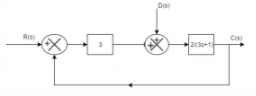Solution:

Explanation: Y(s)/D(s)=2/3s+1/1+3*(2/3s+1)=2/3s+7.

QUESTION: 4

The closed loop gain of the system shown in the given figure is :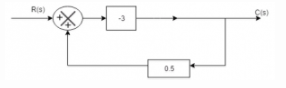Solution:

Explanation: C(s)/R(s)=-3/1+3/2=-6/5.

QUESTION: 5

The advantage of block diagram representation is that it is possible to evaluate the contribution of each component to the overall performance of the system.

Solution:

Explanation: The advantage of the block diagram is that it is possible to get the contribution of each block to the overall performance of the system.

QUESTION: 6

The overall transfer function from block diagram reduction for cascaded blocks is :

Solution:

Explanation: Gain of block get multiplied when they are cascaded where cascaded means that the blocks are in series combination with no summer in between.

QUESTION: 7

The overall transfer function of two blocks in parallel are :

Solution:

Explanation: The gains get added as the blocks are connected in parallel with the summer in between and they are connected with the same sign.

QUESTION: 8

Transfer function of the system is defined as the ratio of Laplace output to Laplace input considering initial conditions________

Solution:

Explanation: By definition transfer function is the ratio of the laplace output to the input but the initial conditions mainly the stored energy is zero.

QUESTION: 9

In the following block diagram, G1=10/s G2=10/s+1 H1=s+3, H2=1. The overall transfer function is given by :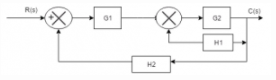Solution: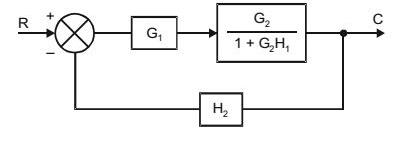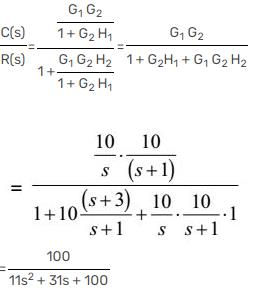QUESTION: 10

Oscillations in output response is due to :

Solution:

Explanation: Oscillations are the unwanted sinuoidal signals with high gain in positive feedback and s the damping factor is absent in the positive feedback system entirely oscillations are present.